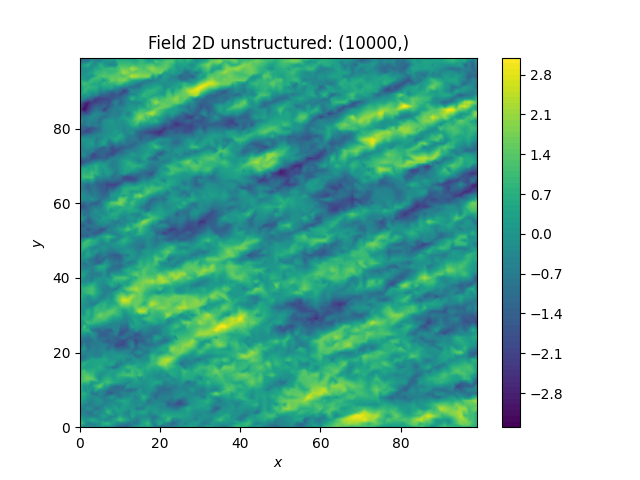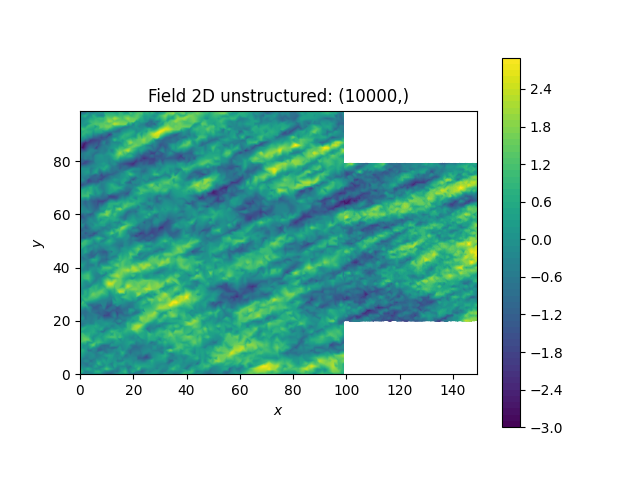# Merging two Fields¶

We can even generate the same field realisation on different grids. Let’s try to merge two unstructured rectangular fields.

```import numpy as np
import gstools as gs

# creating our own unstructured grid
seed = gs.random.MasterRNG(19970221)
rng = np.random.RandomState(seed())
x = rng.randint(0, 100, size=10000)
y = rng.randint(0, 100, size=10000)

model = gs.Exponential(dim=2, var=1, len_scale=[12, 3], angles=np.pi / 8)
srf = gs.SRF(model, seed=20170519)
field1 = srf((x, y))
srf.plot()
```But now we extend the field on the right hand side by creating a new unstructured grid and calculating a field with the same parameters and the same seed on it:

```# new grid
seed = gs.random.MasterRNG(20011012)
rng = np.random.RandomState(seed())
x2 = rng.randint(99, 150, size=10000)
y2 = rng.randint(20, 80, size=10000)

field2 = srf((x2, y2))
ax = srf.plot()
ax.tricontourf(x, y, field1.T, levels=256)
ax.set_aspect("equal")
```The slight mismatch where the two fields were merged is merely due to interpolation problems of the plotting routine. You can convince yourself be increasing the resolution of the grids by a factor of 10.

Of course, this merging could also have been done by appending the grid point `(x2, y2)` to the original grid `(x, y)` before generating the field. But one application scenario would be to generate hugh fields, which would not fit into memory anymore.

Total running time of the script: ( 0 minutes 3.356 seconds)

Gallery generated by Sphinx-Gallery